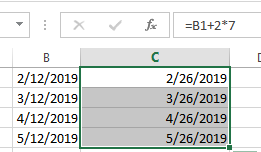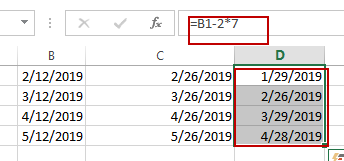# How to Add or Subtract Weeks From a Date in Excel

This post will guide you how to add or subtract weeks to a given date in Excel. How do I add weeks to a date with a formula in Excel. How to subtract weeks to a date in Excel.

## Add Weeks to a Date

Assuming that you have a list of data in range B1:B4, in which contain date values, and you want to add 2 weeks to the date in your range of cells. how to do it. You can use the following formula:

=B1+2*7

Type this formula into cell C1 and press Enter key on your keyboard, and then copying this formula from Cell C1 to range C2:C4.You would notice that all date values in range B1:B4 have been added two weeks.

## Subtract Weeks to a Date

If you want to subtract 2 weeks to all date values in range B1:B4, you can use the following formula:

=B1-2*7

Type this formula into cell D1 and press Enter key on your keyboard, and then copying this formula from Cell D1 to range D2:D4.You would notice that all date values in range B1:B4 have been subtracted two weeks.

Sidebar